Keyah Math Module 4, Level 2

Mathematical Content : Circumference of a circle, geometry, volume of a sphere

#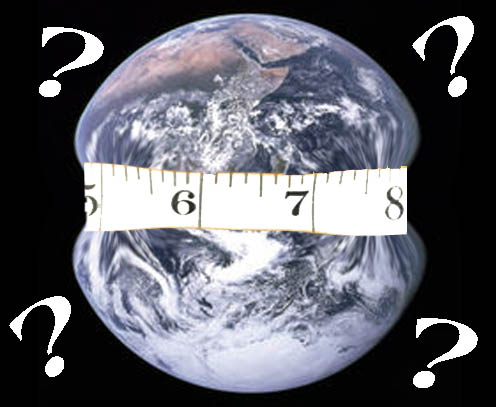Warm up question:
Without leaving this country, how could you figure out how far it is all the way around the World?

Introduction
Around 250 BC, at noon on the day of the summer solstice (when the sun is at its highest point in the Northern Hemisphere) in Syrene, Egypt, sunlight filled the vertical shaft of a well; this indicates that the sun is directly overhead, so a vertical pole would cast no shadow. Eratosthenes, who lived in Alexandria, heard of this from a traveler. So on the same day, different year, he noticed that in Alexandria, some 800 kilometers (km) away, a vertical pole cast a shadow. From these observations, he made two deductions:

A. the earth is curved;

B. found the first estimate for the circumference of the Earth.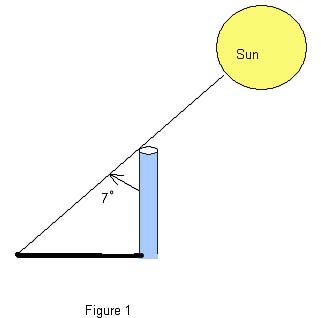The Earth is Spherical
He measured the angle made by the pole and a line joining the tip of the shadow and the top of the pole (see Figure 1) and found the angle to be about 7o. Then he assumed that light rays from the sun to the Earth were essentially parallel since the sun was so far away and the Earth was so small relative to the sun. From this, and his observations in Alexandria and Syrene, he concluded that the Earth must be curved (see Figure 2), and therefore must be spherical.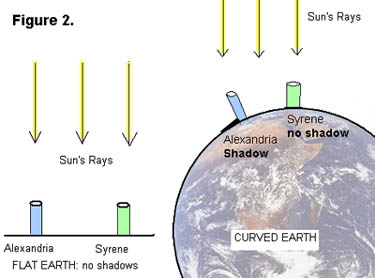Using Math to Find the Circumference of the Earth
Next, he used all this information to obtain the first nearly accurate estimation of the circumference of the Earth. Here’s how: In the (not-to-scale) Figure 3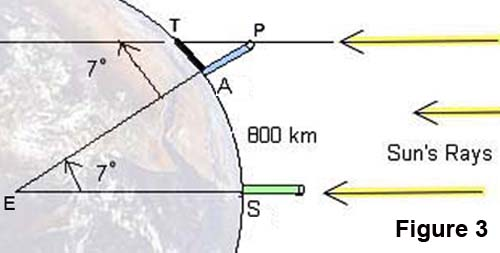A denotes the base of the pole in Alexandria;
S the base of a pole in Syrene;
T the tip of the shadow cast by the pole in Alexandria;
P the top of the same pole;
E the center of the Earth.

Angle APT was measured to be 7o, so by Euclidean geometry interior angles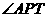and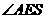are equal, thus angle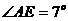.

There are 360o in a complete circle, so the portion of the circumference of the Earth between A and S is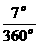, which is approximately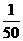(or,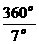is approximately 50).

The distance from Alexandria to Syrene is 800 km, so he concluded that the circumference of the Earth must be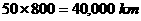!

This estimate is very close to modern accurate measurements, so Eratosthenes gets credit for the first calculation of the size of the Earth.

We can get a slightly different answer if we compute more accurately: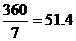, so if we multiply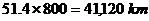Questions

 Notation: Some formulas you’ll need (r = radius of circle / sphere) Circumference of a Circle: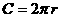Volume of a Sphere: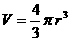Question 1: What is the radius of the Earth? Use Eratosthenes’ estimate for the circumference of the Earth to find its radius. (Round your answer to 1 decimal place.)
 Question 2: What is the volume of the Earth? Use your answer to Question 1 to compute the volume of the Earth. (Round your answer to 3 decimal places.)

Here are some follow-up exercises:

 Study Eratosthenes’ method so you completely understand it. There is a follow-up module to this one: it shows how to repeat this activity using locations in Arizona and suggests that you write a module using places in New Mexico, or elsewhere.

This material is based upon work supported by the National Science Foundation under Grant GEO-0355224. Any opinions, findings, and conclusions or recommendations expressed in this material are those of the authors and do not necessarily reflect the views of the National Science Foundation.